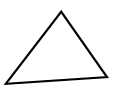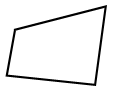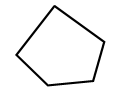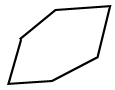# Plane figure

A plane figure is a geometric figure that has no thickness. It lies entirely in one plane. Below are examples of different types of plane figures.

A plane figure can be composed of line segments, curves, or a combination of the two. Plane figures are often categorized as open or closed

## Open

The segments or curves of an open figure are not always connected. At least one endpoint of one side is not connected to the rest. Line segments, curves, angles, and many other shapes are open.

## Closed

The line segments or curves forming a closed figure are continuous, meaning there is no break. The lines/curves must also form a closed region to be considered a closed shape. A closed figure has area and perimeter. The following are a few examples.

Closed figures can be further categorized into polygonal plane figures and non-polygonal plane figures.

### Polygonal plane figures

A polygon is a closed plane figure formed only using line segments. The line segments are called sides. A polygon has at least three sides. There are many classifications of polygons; they are often classified based on the number of sides in the polygon.

Polygon# of sidesShape
Triangle3Quadrilateral4Pentagon5Hexagon6An n-sided polygon is called an n-gon.

### Non-polygonal plane figures

If any side or part of a plane figure is curved (not straight) it is not a polygon. The two most common types of non-polygonal plane figures we see in Geometry are a circle and an ellipse.

#### Circle

A circle is a plane figure formed by the set of all points in a plane that are equidistant from a given, fixed point, called the center.

#### Ellipse

An ellipse is a plane figure in the shape of an oval. We usually think of it as looking like a "flattened" or "stretched" circle. The figure below shows two ellipses.

While the circle and the ellipse are the most common non-polygonal plane figures we see in Geometry, there are many others. As long as any side or part of a plane figure is curved, it is a non-polygonal plane figure.